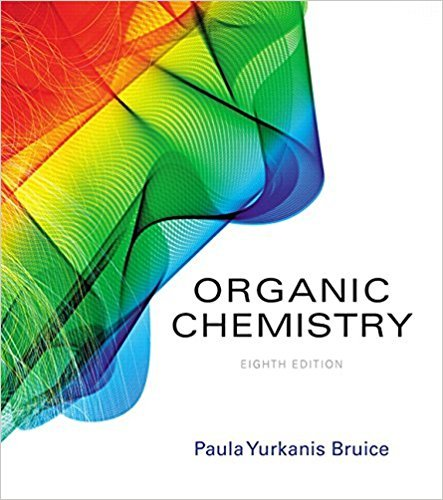×
×

# Solutions for Chapter 2.5: How to Determine the Position of Equilibrium## Full solutions for Organic Chemistry | 8th Edition

ISBN: 9780134042282Solutions for Chapter 2.5: How to Determine the Position of Equilibrium

Solutions for Chapter 2.5
4 5 0 353 Reviews
26
1
##### ISBN: 9780134042282

This expansive textbook survival guide covers the following chapters and their solutions. Since 4 problems in chapter 2.5: How to Determine the Position of Equilibrium have been answered, more than 35159 students have viewed full step-by-step solutions from this chapter. Organic Chemistry was written by and is associated to the ISBN: 9780134042282. This textbook survival guide was created for the textbook: Organic Chemistry, edition: 8. Chapter 2.5: How to Determine the Position of Equilibrium includes 4 full step-by-step solutions.

Key Chemistry Terms and definitions covered in this textbook
• alkali metals

Members of group 1A in the periodic table. (Section 7.7)

• alkylation

A reaction that achieves the installation of an alkyl group. For example, an SN2 reaction in which an alkyl group is connected to an attacking nucleophile.

• Cis

A prefi x meaning on the same side

• Clemmensen reduction

Reduction of the C"O group of an aldehyde or ketone to a CH2 group using Zn(Hg) and HCl

• common-ion effect

A shift of an equilibrium induced by an ion common to the equilibrium. For example, added Na2SO4 decreases the solubility of the slightly soluble salt BaSO4, or added NaF decreases the percent ionization of HF. (Section 17.1)

• coordination number

The number of adjacent atoms to which an atom is directly bonded. In a complex the coordination number of the metal ion is the number of donor atoms to which it is bonded. (Sections 12.37 and 24.2)

A polymer in which neighboring chains are linked together, for example, by disulfide bonds.

• crystal lattice

An imaginary network of points on which the repeating motif of a solid may be imagined to be laid down so that the structure of the crystal is obtained. The motif may be a single atom or a group of atoms. Each lattice point represents an identical environment in the crystal. (Section 12.2)

• elastomers

• enantiomer

A nonsuperimposable mirror image.

• Equatorial bond

A bond to a chair conformation of cyclohexane that extends from the ring roughly perpendicular to the imaginary axis through the center of the ring; a bond that lies roughly along the equator of a cyclohexane ring

• equilibrium

For a reaction, a state in which there is no longer an observable change in the concentrations of reactants and products.

• fishhook arrow

A curved arrow with only one barb, indicating the motion of just one electron (also see Sect. 11.1).

• heterogeneous alloy

An alloy in which the components are not distributed uniformly; instead, two or more distinct phases with characteristic compositions are present. (Section 12.3)

• Keto-enol tautomerism

A type of isomerism involving keto (from ketone) and enol tautomers

• normal melting point

The melting point at 1 atm pressure. (Section 11.6)

• nuclear binding energy

The energy required to decompose an atomic nucleus into its component protons and neutrons. (Section 21.6)

• reducing agent

A compound that reduces another compound and in the process is itself oxidized. Sodium borohydride and lithium aluminum hydride are reducing agents.

• Shielding in NMR

Also called diamagnetic shielding; the term refers to the reduction in magnetic fi eld strength experienced by a nucleus underneath electron density induced to circulate when the molecule is placed in a strong magnetic fi eld.

• symmetric stretching

In IR spectroscopy, when two bonds are stretching in phase with each other.

×